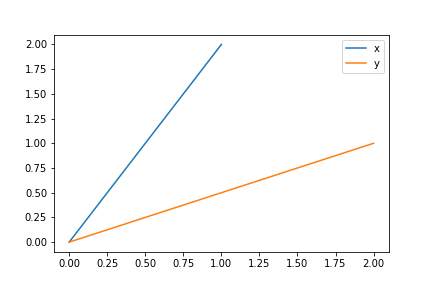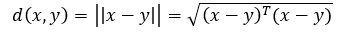#2019 iT 邦幫忙鐵人賽DAY 11
0

## Day 11-機器學習與數學天天玩-PCA-Transformation of Vectors in Spaces: Dot Product Part 1We are now in the PCA journey, station 2.

1. Statistical Introduction
2. Transformation of Vectors in Spaces
2. Orthogonal ProjectionLike the picture above, we want to know the difference between x and y. There are two ways for us to inspect, distance between x and y, and the angle between x and y. When it comes to the distance, the formula is as below:We firstly make x - y, and then have the transpose of (x-y) time (x-y), and then have it be a root square value.

``````import numpy as np

x = np.array([1, 2])
y = np.array([2, 1])

def distance(x, y):

distance = ((x-y)@(x-y))**0.5

return distance

distance(x, y)
``````